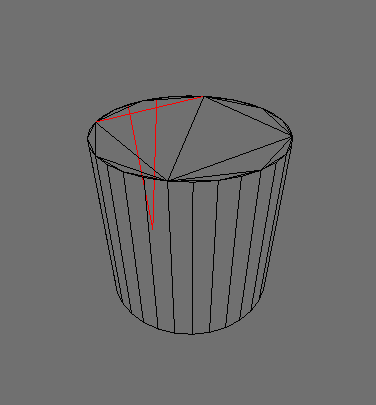# Finding joined vertices

I somehow need to find out which vertices are joined. I need to know their ids.

Let’s say that there is an object with 8 vertices. I need a script that checks them all(or checks whatever needed) and finds out if they’re connected. If they are, than it appends both of connected vertex ids as a list to a larger list.

``````
list.append([vertex1, vertex2])

``````

How can I make such a script?

P.S. I need this to be done only for a single initial frame.

You need to traverse the polygons and associate vertices within polygons as belonging to a face. The edges are defined in cw or anti-cw order (can’t remember which). However, the BGE API is a nightmare - you’ll get new vertex object references if you call getVertex with the same ID, so to only store unique vertex references, you should first check if they’ve been accessed before (e.g):

``````
def get_vertex(mesh, index, mapping, mat_id=0):
try:
return mapping[index]
except KeyError:
mapping[index] = mesh.getVertex(mat_id, index)
return mapping[index]

# Test code here
id_to_vertex = {}
vert1 = get_vertex(mesh, 0, id_to_vertex)
vert1_b = get_vertex(mesh, 0, id_to_vertex)

vert_1_c = mesh.getVertex(0, 0)
assert vert1 is vert1_b
assert vert1 is vert1_c, "Looks like successive calls to getvertex return new instances!"

``````

You also have to watch out for the fact that you’ll get split vertices according to certain rules (in the bge api docs)
The process to handle all this looks something like:

1. Always use the above method to get vertices from the mesh
2. Determine the edge in clockwise/anticlockwise order from the mesh polygons
3. Build an edge mapping by associating each vertex in each polygon with the next N vertices in the polygon (v0=[v1,v2,v3], v1=[v2,v3,v0], v2=[v3,v0,v1] etc)
4. Use a KDTree with a very fine tolerance to find split vertices, and choose one of them consistently to be the “actual” vertex (if you’re only using positions. If you want to be able to edit these later on … you’d need to just store them all in a consistent manner)
5. Depending on if you just want to read the positions, or be able to modify them, either replace all split vertex references with the chosen vertex replacement in step 4, or be more clever and create proxy vertices which handle all N split vertices etc…

What a pain!

Uh… I almost don’t understand anything of this.
Maybe somebody can just create me the whole script which gets me the edge list?

mesh.blend (497 KB)

``````
from mathutils.kdtree import KDTree
from itertools import cycle

def get_vertex(mesh, index, mapping, mat_id=0):
try:
return mapping[index]
except KeyError:
mapping[index] = mesh.getVertex(mat_id, index)
return mapping[index]

def get_polygons(mesh):
num_polygons = mesh.numPolygons
for i in range(num_polygons):
yield mesh.getPolygon(i)

def get_split_to_grouped_vertices(mesh_vertices):
# Build KDTree
kdtree = KDTree(len(mesh_vertices))
for i, vertex in enumerate(mesh_vertices):
kdtree.insert(vertex.XYZ, i)

kdtree.balance()

tolerance = 1e-5
visited_vertices = set()

split_to_group = {}

# Find near vertices (split vertices) and non split vertices
for vertex in mesh_vertices:
if vertex in visited_vertices:
continue

result = kdtree.find_range(vertex.XYZ, tolerance)
group_vertices = tuple([mesh_vertices[i] for (p, i, d) in result])
visited_vertices.update(group_vertices)

for vertex in group_vertices:
split_to_group[vertex] = group_vertices

return split_to_group

def build(cont):
own = cont.owner

mesh = own.meshes

vertex_to_poly = {}
id_to_vertex = {}
polygon_vertices = {}

for polygon in get_polygons(mesh):
polygon_vertices[polygon] = vertices = []

for i in range(polygon.getNumVertex()):
vertex_index = polygon.getVertexIndex(i)
vertex = get_vertex(mesh, vertex_index, id_to_vertex)
# Two-way mappings
vertex_to_poly[vertex] = polygon
vertices.append(vertex)

mesh_vertices = list(vertex_to_poly)
split_to_group = get_split_to_grouped_vertices(mesh_vertices)

# dict(split_to_group=split_to_group, vertex_to_poly=vertex_to_poly, poly_to_vertices=polygon_vertices)

``````

This will find several pieces of useful information, such as the mapping from vertex to face (which can tell you the edges inside that face - find the index of the vertex in the vertex list of the polygons that it belongs to, and the two vertices either side of it (for each found polygon) are connected by edges.

To account for vertex “splitting”, you have to perform this step for each of the split vertices:
See blend

OK! Will this return me a list of edges in final? Cause this code isn’t clear for me…

The inlined code gets you most of the way there (e.g finds duplicated vertices and maps a vertex to it’s polygon (and polygon to polygon vertices))

You still need to use the get_vertex method to get vertices though, otherwise things won’t work. If you need to do lookups of vertices from positions, you can use the KDTree in the code (make it an argument rather than building it inside one of the functions)

After taking a look at your providen .blend, it tends to do almost great. However, it’s still doesn’t seem right.Those are definetly not edges of a single vertex.

By the way - the edges are defined as sets of 2 vertices, right? If so, than I propably just need to know the vertex which the current vertex is joined now with. So the edge can be actually another vertex or vertex id stored in my dictionary referring to the other end of the edge.

However, this still doesn’t work exactly as needed.

### AttachmentsAfter doing some print debugging I understood that this system gets all of the edges that belongs to a certain polygon. However, I need to get a list of edges that all contains single vertex. Is it possible?

The blend should do just that, but there may be a mistake

Hm… Is it supposed to get all the edges that contains the exact vertex? If so, than it possible has a little mistake.